# Day 7-9 – 05-07 Dec 2020 — Recap

A few days ago I finished the Lanes finding module and today I will briefly explain in this post what that was about, and share some of my thoughts regarding the topic.

# Progress

I will attempt to list out A the necessary steps at a high level and detail as much as needed on the most important ones. I should start by stating that for this module, and probably for the subsequent ones too, the libraries I will use are NumPy and OpenCV. NumPy allows for complex multi-dimensional arrays and matrices mathematical operations, and OpenCV is a library of functions aimed at real time computer vision [ref], I use it in this module for image loading, processing and rendering.

The code for this module can be on my GitHub, here.

# Step 0 — Understanding what an image is

It is important mentioning that an image is is a set (list/array) of pixels. Each pixel has its own colour, represented in the RGB (red-green-blue) space.

Given the image above, its dimensions are 2400 x 1600 px, meaning the width is 2,400 pixels across and the height is 1,600 (vertically).

Each pixel is, like stated above, represented by an RGB color value, such as (37,114,184), which is the color of one pixel of the sky in this image. Each number represents the intensity of the color it represents on a scale from 0 to 255. Where 0 means no intensity (black), 255 means high intensity (white). Hence black is (0, 0, 0) and white (255, 255, 255).

In code, an image like such is represented as a multidimensional array of colour codes just like in the examples above. Here is an example of a cut-down version of an example image, where I printed to the console an image read using OpenCV:

It is important to understand the structure of an image, because it will help us better understand how it gets processed.

# Step 1 — Loading and displaying

First off, I’ll have to import NumPy and OpenCV in my app:

import cv2

import numpy as np

As easy as that. I could then use OpenCV across the file as cv2 and NumPy as np.

Loading the image is simple, I placed a sample image in the same directory as my Python file and with just one line of code, using the

image = cv2.imread(‘test_image.jpg’)

To display it, I just use cv2.imshow(“result”, final_image)

# Step 2 — Turning the image greyscale

This step is necessary because, by doing it, we help the program run faster and be more efficient in detecting the lanes, you’ll see why later. The only thing I would add here is a brief explanation of what grayscaling actually is. It turns a pixel RGB color (37,114,184) into just a single number on the 0–255 scale, but it holds only one value. For example, the blue color in the color code above, grayscaled is 112. So on the gray scale, the new color looks like this (355).

But how did I come up with 112 from (37,114,184)? Well that’s simple, it’s just the mean average of the three color intensities (37+114+184)/3. Cool right? So OpenCV does that for each and every pixel in the image input.

Here’s the code and output result:

gray = cv2.cvtColor(image, cv2.COLOR_RGB2GRAY)

# Step 3 — Gaussian Blur

Now a Gaussian Blur/Filter is necessary. This is because It makes it easier for the next step, which is edge detection. Here is the code and the result:

blur = cv2.GaussianBlur(gray, (5, 5), 0)

The OpenCV GussianBlur does what is says on the box, adds a blur to the given image. The second argument is the number of pixels (width, height) it takes the average colour from. And so to add the blur it takes the average color for the surrounding 5 pixels on all sizes, resulting in this:

# Step 4 — Canny Detection

This step is really cool. As the heading says, it detects all the edges. It does this by applying a threshold over the colors of the image. This threshold is meant to tell the algorithm what color intensities are considered acceptable. Here is the code:

canny = cv2.Canny(blur, 50, 150)

The last 2 arguments are the thresholds. 50 means that colours with intensities lower than 50 are turned off (0/black), and colours higher than 150 are turned white (255). So everything becomes literally black and white:

# Step 5 — Defining area

This step narrows the view field to allow for better lines detection. It is not worth asking the program to find the lanes in the fields, when we know it’ll always find them at certain positions. This could be problematic for generalised use, but this module is meant to provide an introduction to lanes detection. Later, more advanced algorithms will be used to detect lanes.

def region_of_interest(image):
# height is the first axis from the shape property
height = image.shape
polygons = np.array([
[(319, height), # point B
(982, height), # point C
(554, 258)] # point A
])
# draw a mask of the same shape as the image
mask = np.zeros_like(image) # zeros is color black
# fill the polygon with our triangle, bg color of white
cv2.fillPoly(mask, polygons, 255)
return mask

Here I define a function to do this, it takes an image input, we can work with the original image at this step, we’re only making a mask in preparation for what’s to come.

To better understand the code, here is a graph I generated using pyplot:

That is the canny image on a graph, to help us see its dimensions. As you can see, the processed image height is 704px and width ~1279px. To get the height, we need to access the shape property of the image. Which is uni-dimensional array that looks like this:

(704, 1279, 3)

Where 704 is the height and 1279, you guessed it, the width in our case (3 is the depth).

So we need to draw a triangle as a mask, like the one on the left.

The three points, A, B and C are the ones in the code (coordinates). We use those coordinates to make an array that we pass in to OpenCV fillPoly function a bit down. Before then we do one more thing. We create a maks. And we do this by creating an exact copy of the image, but all black. np.zeros_like creates an identical array such as the image, but with zeros instead of all the colour codes (zero represents no intensity therefore color black, remember?). Then we use the mask along with our new array to fill the mask with the polygon drawn. The result so far looks like this:

# Step 6 — Bitwise and

This step below creates a true white triangle over a black background with the same exact dimensions of the original image. Now the mask is passed into a function cv2.bitwise_and(mask, image) along with the original image, to create this: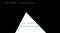Figure 1

But what is bitwise_and you might ask. It takes in two arguments, the mask and the original image (we’re only working with arrays, so when I say image, that’s what I mean, not the actual image, but the rgb colour representation).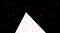Figure 2

Then it applies an AND operator over each of the elements of the array compared to the counterpart. In Figure 1, you can see the color code and their representation in binary. Color black is represented by number 0, which translated to binary is still 0. Color white is 255, which represented in binary, is 11111111. In Figure 2, I applied my Microsoft Paint skills to show you how the function turns the colours in binary. Then the last part of this step is to apply an end over each element of the array compared to the canny image. Under the hood it looks something like this:

Given color 75 (dark grey) in binary is 1001011. 0 AND 1001011 = 0. Therefore if that color grey is present in the areas masked as black, the resulted color is always going to be black.

However, if color 75 (1001011) is compared against the white triangle the calculation looks like this: 1 AND 1001011 = 1. This means that everything inside of the triangle stays unchanged, whereas the areas outside, gets turned to black, so this is what we’ve achieved in this step:

# Step 7 — Hough Lines

Now that we have our lanes simplified and clear, we can use an OpenCV function HoughLinesP, to draw the lines that can be later used as guidance for driving in between them, as follows:

cv2.HoughLinesP(cropped_image, 2, np.pi/180, 100, np.array([]), minLineLength=40, maxLineGap=5)

I should begin this step by explaining what Hough space is. Every line in the Cartesian space is represented by a point in the Hough space, courtesy of the following linear equation:

y = mx + b

Where m is called slope and b is y-intercept.

Let’s start with m. This is the rate at which our line rises or falls. So its definition is this:

m = vertical change / horizontal change

or

m = (y2-y1)/(x2–x1)

b is the location the line hits the y-axis, hence why it’s called y-intercept.

I would suggest watching a YT video about this, it’s way easier to understand when you have visuals.

Moving on, a dot (point) in the Cartesian space is represented by a line in the Hough space. This is because an infinite number of lines can pass through a dot. And so an infinite number of points in Hough, form a line.

The bottom line is this: If multiple points in the Cartesian space intersect in Hough, it means they can form a line, like in the figure above.

So getting back to our function:

cv2.HoughLinesP(cropped_image, 2, np.pi/180, 100, np.array([]), minLineLength=40, maxLineGap=5)

The first argument is the cropped image generated in Step 6. The second argument is the bin size used to decide whether the line detected is worthy of being considered a lane line. The third argument is the precision in degrees. Argument 4 is a threshold that specifies the minimum number of intersections for a line to be considered. Argument 5 is just an empty array passed in. The sixth argument is the lenght of the line in px accepted in the output. And the last one is the max line gap: the maximum distance in px between segmented which we’ll allow to be connected into a single one.

Finally, still as part of this step I created a function to draw the lines on a black background:

def display_lines(image, lines):
line_image = np.zeros_like(image) # draw a black background
if lines is not None: # if there are any Hough lines detected
for line in lines: # iterate though each of them
“””
Note: each line in lines is a 2D array reshape turns it into a 1D
array or better yet, for each line, save the coordinates into their
respective variables.
“””
x1, y1, x2, y2 = line.reshape(4)
“””
Use the coordinates to draw the line.
arg1: the background
arg2: Starting point of the line segment (x and y coordinates)
arg3: Ending point of the line segment (x and y coordinates)
arg4: Line color
arg5: Line thickness
“””
cv2.line(line_image, (x1, y1), (x2, y2), (255 , 0, 0), 10)
return line_image

We do this by firstly drawing up a black background, nothing new here. Then iterating through the array of lines detected previously. I then take the line (on each iteration) and using the reshape function, I save its coordinates into 4 local variables (x1, x2, y1, y2). The following part of the code is pretty self explanatory, I draw the line over the black background generated above the while loop. Therefore we end up with a bunch of lines like so: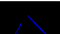Hough lines on top of a black background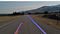Hough lines over the original image

# Step 8 — Optimization

At this last step, I created a function to calculate the average slope and y-intercept to draw a single line for each side.

At first we define a couple of arrays to hold the slope and y-intercept for each side:

left_fit = []
right_fit = []

Then we iterate over each line defined at Step 7:

for line in lines:

Again, we take the coordinates of the line (through each iteration):

x1, y1, x2, y2 = line.reshape(4)

Then we calculate the slope and y-intercept values (given the coordinates of the line) using the following function:

parameters = np.polyfit((x1, x2), (y1, y2), 1)

That returns an array where the first item is the slope and the second the y-intercept, we store them as follows:

slope, intercept = parameters, parameters

Then we decide if the line pertains to the left or right side. If the slope of a line is negative, it means it pertains to the left side. Again, a video is better at explaining this than myself. We apply this logic bellow:

if slope < 0:
left_fit.append([slope, intercept])
else:
right_fit.append([slope, intercept])

When the iteration is complete, we end up with 2 arrays containing a bunch of slopes and y-intercepts for each of the lines detected. Then outside the iteration, we calculate the average slope and y-intercept for each of the sides, like so:

left_fit_average = np.average(left_fit, axis=0)
right_fit_average = np.average(right_fit, axis=0)

This is pretty easy to understand, it calculates the average values on the axis 0, meaning vertically on the arrays given: [[1, .78], [1.34, .12]] is (1 + 1.34)/2 and (.78 + .12)/2.

Once we have these we define the left and right line like so:

left_line = make_coordinates(image, left_fit_average)
right_line = make_coordinates(image, right_fit_average)

The make_coordinates function is created by me and it just turns the slope and y-intercept back into coordinates using y = mx + b.

slope, intercept = line_parameters
y1 = image.shape
y2 = int(y1*3/5)
x1 = int((y1-intercept)/slope)
x2 = int((y2 -intercept)/slope)
return np.array([x1, y1, x2, y2])

Firstly we grab the slope and y-intercept for the line passed in. Then we take the y1 (which is just the image height). Then we calculate the y2 (which is approximately towards the middle of the image, where the road ends). Then x1 and x2 are calculated using the Hough inverse formula: x= (y-b)/m. finally we combine all those coordinates into an array that we draw on top of our image to get this: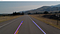Averaged Hough lines on top of the original image

As you can see above, we have two clean-cut lines on top of the original image.

# Step 9 — Video functionality

The final step is to allow lanes detection for a video. But we know that a video is just a bunch of images bound together. So we can accommodate for video input with very little effort.

cap = cv2.VideoCapture(‘test2.mp4’)

I place a video in the same directory as my python file. Then reference it using the above function.

while cap.isOpened():
_, frame = cap.read()
if render(frame, 1):
break
cap.release()
cv2.destroyAllWindows()

Then all I do is iterate over each frame and call the function render, which is all the above functionality nicely refactored for reusability.

Our end result, is this:

As you can see, the lines are wobbly and sometimes intermittent, but remember, this module was meant to getting me used to edge detection, image processing, Hough calculations, etc.

# Thoughts

This module was a pretty nice introduction in the power of NumPy and OpenCV. Learnt a lot, and definitely expanded my knowledge in terms of Computer Vision (from 0 to 1 at least).

# Next

The next module is now Perception. Looking forward to it, I’ll come back with updates tomorrow.

Programmer as a job, curious as a passion